# RD Sharma Class 9 Solutions Chapter 5 Factorisation of Algebraic Expressions Ex 5.2

## RD Sharma Class 9 Solutions Chapter 5 Factorisation of Algebraic Expressions Ex 5.2

These Solutions are part of RD Sharma Class 9 Solutions. Here we have given RD Sharma Class 9 Solutions Chapter 5 Factorisation of Algebraic Expressions Ex 5.2

Other Exercises

Factorize each of the following expressions:
Question 1.
p3 + 27
Solution:
We know that a3 + b3 = (a + b) (a2 – ab + b2)
a3 – b3 = (a – b) (a2 + aft + b2)
p3 + 21 = (p)3 + (3)3
= (p + 3) (p2– p x 3 + 32)
= (p + 3) (p2 – 3p + 9)

Question 2.
y3 + 125
Solution:
y3 + 125 = (p)3 + (5)3
= (p + 5) (p2 – 5y + 52)
= (P + 5) (p2 – 5y + 25)

Question 3.
1 – 21a3
Solution:
1 – 21a3 = (1)3 – (3a)3
= (1 – 3a) [12 + 1 x 3a + (3a)2]
= (1 – 3a) (1 + 3a + 9a2)

Question 4.
8x3y3 + 27a3
Solution:
8x3y3 + 27a3
= (2xy + 3a) [(2xy)2 – 2xy x 3a + (3a)2]
= (2xy + 3a) (4x2y – 6xya + 9a2)

Question 5.
64a3 – b3
Solution:
64a3 – b3 = (4a)3 – (b)3
= (4a – b) [(4a)2 + 4a x b + (b)2]
= (4a – b) (16a2 + 4ab + b2)

Question 6.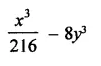Solution: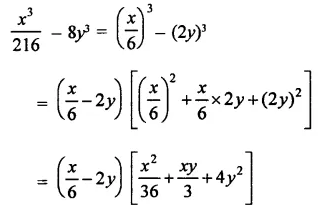Question 7.
10x4– 10xy4
Solution:
I0x4y- 10xy4 = 10xy(x3 -y3)
= 10xy(x – y) (x2 + xy + y2)

Question 8.
54x6y + 2x3y4
Solution:
54 x6y + 2x3y4 = 2x3y(27x3 + y3)
= 2x3y[(3x)3 + (y)3]
= 2x3y(3x + y) [(3x)2 -3x x y + y2]
= 2x3y(3x + y) (9x2 -3xy + y2)

Question 9.
32a3 + 108b3
Solution:
32a3 + 108b3
= 4(8a3 + 27b3) = 4 [(2a)3 + (3 b)3]
= 4(2a + 3b) [(2a)2 – 2a x 3b + (3b)2]
= 4(2a + 3b) (4a2 – 6ab + 9b2)

Question 10.
(a – 2b)3 – 512b3
Solution:
(a – 2b)3 – 512b3
= (a – 2b)3 – (8b)3
= (a – 2b- 8b) [(a – 2b)2 + (a – 2b) x 8b + (8b)2]
= (a – 10b) [a2 + 4b2 – 4ab + 8ab – 16b2 + 64b2]
= (a – 10b) (a2 + 4ab + 52b2)

Question 11.
8x2y3 – x5
Solution:
8x2y3 – x5 = x2(8y3 – X3)
= x2(2y)3 – (x)3]
= x2[(2y – x) (2y)2 + 2y x x + (x)2]
= x2(2y – x) (4y2 + 2xy + x2)

Question 12.
1029 -3x3
Solution:
1029 – 3X3 = 3(343 – x3)                       ‘
= 3 [(7)3 – (x)3]
= 3(7 – x) (49x + 7x + x2)

Question 13.
x3y3+ 1
Solution:
x3y3 + 1 = (xy)3 + (1)3
= (xy + 1) [(xy)2 – xy x 1 + (1)2]
= (xy + 1) (x2y2 – xy + 1)
= (xy + 1) (x2y – xy + 1)

Question 14.
x4y4 – xy
Solution:
x4y4 – xy = xy(x3y3 – 1)
= xy[(xy3-(1)3]
= xy (xy – 1) [x2y2 + 2xy + 1]

Question 15.
a3 + b3 + a + b
Solution:
a3 + b3 + a + b
= (a + b) (a2 – ab + b2) + 1 (a + b)
= (a + b) (a2 – ab + b2 + 1)

Question 16.
Simplify: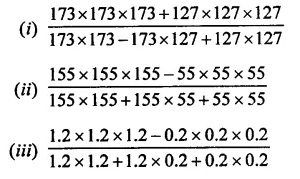Solution: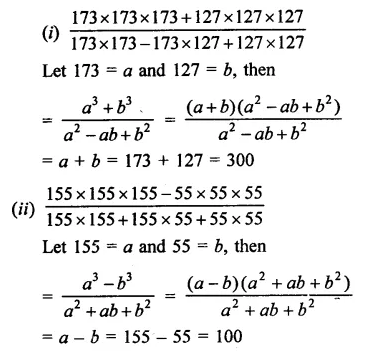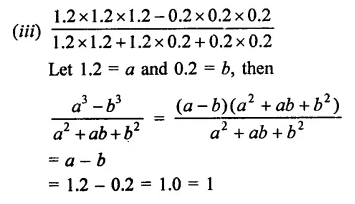Question 17.
(a + b)3 – 8(a – b)3
Solution:
(a + b)3 – 8(a – b)3
= (a + b)2 – (2a – 2b)3
= (a+ b – 2a + 2b) [(a + b)2 + (a + b) (2a-2b) + (2a – 2b)2)]
= (3b – a) [a2 + b2 + 2ab + 2a22ab + 2ab – 2b2 + 4a2 – 8ab + 4b2]
= (3b – a) [7a2 – 6ab + 3b2]

Question 18.
(x + 2)3 + (x- 2)3
Solution:
(x + 2)3 + (x – 2)3
= (x + 2 + x – 2) [(x + 2)2 – (x + 2) (x – 2) + (x – 2)2]
= 2x [x2 + 4x + 4 – (x2 + 2x – 2x – 4) + x4x + 4]
= 2x[x2 + 4x + 4- x2-2x + 2x + 4+ x2– 4x + 4]
= 2x[x2 + 12]

Question 19.
x6 +y6
Solution:
x6 + y= (x2)3 + (y2)3
= (x2 + y2) [x4 – x2y2 + y4]

Question 20.
a12 + b12
Solution:
a12 + b12 = (a4)3 + (b4)3
= (a4 + b4) [(a4)2 – a4b4 + (b4)2]
= (a4 + b4) (a8 – a4b4 + b8)

Question 21.
x3 + 6x2 + 12x + 16
Solution:
x3 + 6x2 + 12x + 16
= (x)3 + 3.x2.2 + 3.x.4 + (2)3 + 8           {∵ a3 + 3a2b + 3ab2 +b3 = (a + b)3}
= (x + 2)3 + 8 = (x + 2)3 + (2)3
= (x + 2 + 2) [(x + 2)2 – (x + 2) x 2 + (2)2] {∵ a3 + b2 = (a + b) (a2 – ab + b2}
= (x + 4) (x2 + 4x + 4 – 2x – 4 + 4)
= (x + 4) (x2 + 2x + 4)

Question 22.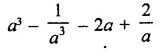Solution: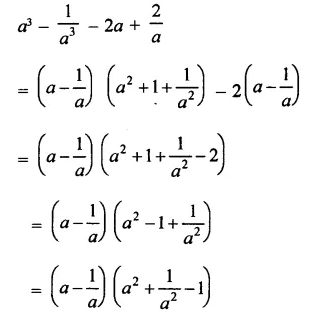Question 23.
a3 + 3a2b + 3ab2 + b3 – 8
Solution:
a3 + 3a2b + 3ab2 + b3 – 8
= (a + b)3 – (2)3
= (a + b -2)[(a + b)2 + (a +b)x2 + (2)2]
= (a + b-2) (a2 + b2 + 2ab + 2a + 2b + 4)
= (a + b – 2) (a2 + b2 + 2ab + 2(a + b) + 4]
= (a + b – 2) [(a + b)2 + 2(a + b) + 4}

Question 24.
8a3 – b3 – 4ax + 2bx
Solution:
8a3 – b3 – 4ax + 2bx
(2a)3 – (b)3 – 2x(2a – b)
= (2a-b)[(2a)2 + 2a x b + (b)2]- 2x(2a-b)
= (2a – b) [4a2 + 2ab + b2] – 2x(2a – b)
= (2a – b) [4a2 + 2ab + b2 – 2x]

Hope given RD Sharma Class 9 Solutions Chapter 5 Factorisation of Algebraic Expressions Ex 5.2 are helpful to complete your math homework.

If you have any doubts, please comment below. Learn Insta try to provide online math tutoring for you.